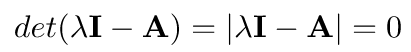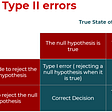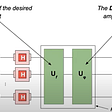# The Variational Quantum Eigensolver, Implemented with IBM’s Qiskit

If you dabbled in quantum algorithms, you’ve probably heard of the term ‘variational algorithms’ being thrown around a lot.

Well, here’s why.

The long story short version is that our quantum hardware isn’t nearly as good as it should be (ie, lots of noise, decoherence…) and so, the algorithms that we run on it are only going to as accurate as they are ‘resilient’ to all this noise the quantum computer throws as it.

So, in the meantime, we’re using quantum computer terms as the NISQ (noisy intermediate-scale quantum) era quantum devices. They don’t have a huge amount of qubits and also don’t have an amazingly low error rate.

So, since 2012, researchers have developed (and continue to develop) hybrid quantum-classical algorithms called variational algorithms. These algorithms offload most of the ‘computing’ work to the classical computer and only have the quantum computer compute the ‘quantum’ part. Additionally, these variational algorithms are also more robust to error, meaning they still perform well despite all the noise.

In a nutshell, without these variational algorithms, we’d be sitting around waiting for hardware to develop. So hooray for them!

Some common variational algorithms:

• Variational Quantum Eigensolver (VQE)
• Quantum Adiabatic Optimization Algorithm (QAOA)
• Quantum Neural Networks (QNN)
• Quantum Support Vector Machines (QSVM)
• Variational Quantum Classifier (VQC)
• Quantum GAN (QGAN)

These algorithms have endless applications, with some of the most promising ones in computational chemistry, optimization problems, and machine learning (as some of the names of the above algorithms suggest)!

So, today, we’re going to briefly delve into the variational quantum eigensolver (VQE) by 1) motivating the intuition behind it and go over some quick math 2) implement it using IBM’s Qiskit package!

Specifically, we’ll apply it to a computational chemistry problem of finding the ground state energy of a H2 molecule.

# How the heck VQEs work

It’s really just a fancy / very elegantly designed feedback loop that sticks your quantum computer with your classical computer together. No, really (that wasn’t sarcasm).

Actually, fancy and (very) elegant are pretty accurate descriptions in my opinion because there are a lot of beautiful nuances in the algorithm that allows it to perform so well despite all the noise in these NISQ era devices.

## The theory (and all the beautiful nuances)

So the steps are of a general VQE algorithm are as follows:

1. Map the problem that you want to solve to finding the ground state energy of a Hamiltonian (ie, a molecule problem or some cost function)
2. Prepare a trial state with some collection of parameters
3. Run that through the parameterized quantum circuit
4. Measure expectation values of Hamiltonian (by measuring each of the Pauli strings or the ‘observables’ of the Hamiltonian)
5. Calculate the ‘energy’ corresponding to the trial state by summing up all the measurements in step #4
6. Update all the parameters via some optimization algorithm (ie, some form of gradient descent)
7. Now you have the first iteration of the trial state with some new parameters to feedback into step 2 and just repeat everything all over again until you get the lowest possible energy.

Steps 2–4 happen on the quantum computer and steps 5–6 happen on the classical computer. The algorithm is iterative, meaning it repeats itself and feeds it as many times as necessary through this awesome feedback loop until you reach some convergence criterion (for example, the algorithm stops the difference between iterative energies is 10^-6).

Here is the algorithm visualized. Note that QPU refers to the quantum processing unit (the quantum computer) and CPU refers to the classical processing unit (the classical computer).

Woohoo! You now have an intuition of how the algorithm works. Now, onto the math behind it.

## The math behind VQE

The ultimate goal of this algorithm is to find the lowest ‘energy’ of the specified Hamiltonian that defines our problem. That translates to the lowest eigenvalue associated with a given Hermitian matrix, which corresponds to the ground state energy.

BTW, the Hermitian matrix is just a matrix that is equal to its own conjugate transpose (ie, turning an m times n matrix into an n times m matrix).

The spectral theorem states that the eigenvalues of a Hermitian matrix must be real. And since any measurable quantity must be real, than using Hermitian matrices to describe the specified Hamiltonian for our quantum system (the molecule) is appropriate.

If you’re looking for math that would be more specific to a Hamiltonian with respect to the electron orbitals in the molecule, look here.

So, here’s what we’re doing in VQE.

Our goal: given a state (ie, the vector in the below equation) and a matrix (ie, A as the transformation matrix in the below equation), find the lowest associated eigenvalue (ie, lambda as the eigenvalue in the below equation).

A quick crash course on eigenvalues and matrices:

Notice that on the left side of the above equation, we have matrix multiplication and on the right side, we have scalar multiplication. So, to avoid this awkwardness, we multiple the right side by the identity matrix and then move everything to the left. Then we factor out the vector such that:

However, the only way this is ever possible, wherein a non-zero vector (v), once multiplied by some matrix, can be equal to 0, is if its determinant is 0 as such:A zero determinant is like the ‘squishification’ of the space onto 1 line or 1 point.

The exact value of lamda that allows this to happen is the eigenvalue that we’re looking for.

Now, back to finding the lowest eigenvalue for our problem.

A Hermitian matrix can be described as such:

Then, our expectation value for some quantum state, |ψ⟩, is:

If we plug in the Hermitian with the above representation, we get:

This shows that we can use a linear combination of the eigenvalues of the Hermitian as the weights to represent the expectation value of any observable on any quantum state.

Next, if we bring the variational principle (also known as the variational method), we get this:

It’s because this principle/method states that the expectation value of any wave function will always greater or equal to the minimum eigenvalue associated with H.

This, above, is precisely why the VQE algorithm works.

When the Hamiltonian of a system is represented by the Hermitian matrix, H, the ground state energy of that system is the smallest eigenvalue associated with H.

So, we arbitrarily select for a wave function (called the ansatz) as an initial starting point, or a guess, to 1) approximate the minimum λ_min and 2) calculate its expectation value and finally 3) iteratively update the wave function, we get arbitrarily close to the ground state energy of the Hamiltonian.

Now, let’s implement it!

# Applying VQE to find the ground state energy of H2 using IBM’s Qiskit

#1: Install everything: you’ll need 1) Qiskit 2) pyquante2. To make your life easier, you can just clone the pyquante driver from its Github repository:

`git clone https://github.com/rpmuller/pyquante2.git`

#2: Import all the packages that we’ll need to use

`import numpy as npimport pylabimport copyfrom qiskit import BasicAerfrom qiskit.aqua import aqua_globals, QuantumInstancefrom qiskit.aqua.algorithms import ExactEigensolver, VQEfrom qiskit.aqua.components.optimizers import COBYLAfrom qiskit.chemistry.aqua_extensions.components.initial_states import HartreeFockfrom qiskit.chemistry.aqua_extensions.components.variational_forms import UCCSDfrom qiskit.chemistry.drivers import PySCFDriverfrom qiskit.chemistry.core import Hamiltonian, QubitMappingType`

#3: Define the molecule and configure the interatomic distance (starting point and range)

We also define the 2 algorithms that we’ll be using, VQE and the Exact Eigensolver (since H2 is a really small molecule, we can calculate the exact eigenvalues and compare then with the eigenvalues our VQE computes.

Then, we set all the variables: 1) energies 2) hf_energies (Hartree-Fock energies used to compare our VQE to the Exact Eigensolver energies) and 3) distance, where we’ll store all our computed values in later.

`molecule = 'H .0 .0 -{0}; H .0 .0 {0}'algorithms = ['VQE', 'ExactEigensolver']start = 0.5  # Start distanceby    = 0.5  # How much to increase distance bysteps = 23   # Number of steps to increase byenergies = np.empty([len(algorithms), steps+1])hf_energies = np.empty(steps+1)distances = np.empty(steps+1)eval_counts = np.empty(steps+1)`

#4: Define the VQE algorithm (as in how it’ll iterate).

We first set a loop to run both algorithms (VQE and Exact Eigensolver) as many times as necessary to reach convergence.

We find the Fermionic operator of the molecule with the PySCF driver and then map the Fermionic hamiltonian onto a qubit hamiltonian. Then, we set up 2 parameters, qubit_op and qux_ops to contain we mapped qubit hamiltonian, which will act as the operator for the VQE.

`print('Processing step __', end='')for i in range(steps+1):    print('\b\b{:2d}'.format(i), end='', flush=True)    d = start + i*by/stepsfor j in range(len(algorithms)):        driver = PySCFDriver(molecule.format(d/2), basis='sto3g')        qmolecule = driver.run()        operator =  Hamiltonian(qubit_mapping=QubitMappingType.JORDAN_WIGNER,                                two_qubit_reduction=False)        qubit_op, aux_ops = operator.run(qmolecule)`

Next, we’ll run both VQE and Exact Eigensolvers. We also run the COBYLA optimizer, which will iterate on the algorithm for 1000 more times to increase the accuracy and precision of the outputs.

`if algorithms[j] == 'ExactEigensolver':            result = ExactEigensolver(qubit_op).run()        else: #otherwise, run the VQE (has 3 components: the optimizer, the initial state, and the variational form)            optimizer = COBYLA(maxiter=10000)            initial_state = HartreeFock(qubit_op.num_qubits,                                    operator.molecule_info['num_orbitals'],                                        operator.molecule_info['num_particles'],                                        qubit_mapping=operator._qubit_mapping,                                        two_qubit_reduction=operator._two_qubit_reduction)            var_form = UCCSD(qubit_op.num_qubits, depth=1,                            num_orbitals=operator.molecule_info['num_orbitals'],                            num_particles=operator.molecule_info['num_particles'],                            initial_state=initial_state,                            qubit_mapping=operator._qubit_mapping,                            two_qubit_reduction=operator._two_qubit_reduction)            algo = VQE(qubit_op, var_form, optimizer`

#5: Now we’ll initialize and run both algorithms on IBM’s state vector simulator backend, and store the results in their respective variables.

`result = algo.run(QuantumInstance(BasicAer.get_backend('statevector_simulator'))) # define the variables for our results and print themlines, result = operator.process_algorithm_result(result)energies[j][i] = result['energy']hf_energies[i] = result['hf_energy']if algorithms[j] == 'VQE':    eval_counts[i] = result['algorithm_retvals']['eval_count']    distances[i] = dprint(' --- complete')print('Distances: ', distances) #interatmomic distanceprint('Energies:', energies) # ground state energy calculated by the exact eigensolver and the VQEprint('Hartree-Fock energies:', hf_energies) # energies calculated by the Hartree Fock algorithmprint('VQE num evaluations:', eval_counts)`

This is the output you should get:

`Processing step __ 0 1 2 3 4 5 6 7 8 91011121314151617181920212223 --- completeDistances:  [0.5        0.52173913 0.54347826 0.56521739 0.58695652 0.60869565 0.63043478 0.65217391 0.67391304 0.69565217 0.7173913  0.73913043 0.76086957 0.7826087  0.80434783 0.82608696 0.84782609 0.86956522 0.89130435 0.91304348 0.93478261 0.95652174 0.97826087 1.        ]Energies: [[-1.05515977 -1.07344995 -1.08862234 -1.10109216 -1.11121301 -1.11928813  -1.12557945 -1.13031403 -1.13368986 -1.13588003 -1.13703598 -1.13729068  -1.13676067 -1.13554825 -1.13374316 -1.13142414 -1.12866032 -1.12551235  -1.12203364 -1.11827121 -1.11426669 -1.11005699 -1.10567502 -1.10115032] [-1.05515979 -1.07344997 -1.08862236 -1.10109218 -1.11121303 -1.11928818  -1.12557946 -1.13031404 -1.13368989 -1.13588003 -1.13703601 -1.13729069  -1.13676068 -1.13554825 -1.13374316 -1.13142415 -1.12866032 -1.12551236  -1.12203365 -1.11827123 -1.1142667  -1.11005699 -1.10567503 -1.10115033]]Hartree-Fock energies: [-1.04299627 -1.06069042 -1.07523689 -1.08705022 -1.09648327 -1.1038386 -1.10937726 -1.11332565 -1.11588089 -1.11721511 -1.11747891 -1.11680414 -1.11530625 -1.11308635 -1.11023288 -1.10682319 -1.10292491 -1.09859715 -1.09389166 -1.08885376 -1.08352332 -1.07793547 -1.07212134 -1.06610865]VQE num evaluations: [51. 50. 50. 52. 52. 46. 51. 49. 50. 54. 49. 45. 47. 51. 41. 51. 46. 45. 44. 48. 50. 50. 51. 55.]`

#6: Visualize the results using pylab!

`pylab.plot(distances, hf_energies, label='Hartree-Fock')for j in range(len(algorithms)):    pylab.plot(distances, energies[j], label=algorithms[j])pylab.xlabel('Interatomic distance')pylab.ylabel('Energy')pylab.title('H2 Ground State Energy')pylab.legend(loc='upper right');`

Graph outputted:

Note: you can’t see the orange line (VQE energies) because the green line (ExactEigensolver energies) overlaps directly on top of it! That’s a good sign because it means our VQE algorithm performed quite well.

We can also compare the differences between the exact eigensolver and the 2 other algorithms!

`pylab.plot(distances, np.subtract(hf_energies, energies), label='Hartree-Fock')pylab.plot(distances, np.subtract(energies, energies), label='VQE')pylab.xlabel('Interatomic distance')pylab.ylabel('Energy')pylab.title('Energy difference from ExactEigensolver')pylab.legend(loc='upper left');`

Graph outputted:

Notice that VQE basically has no difference! However, note that it’s partially due to the fact that our molecule (H2) is tiny, meaning the Hamiltonian and the respective eigenvalues that need to be calculated are relatively simple.

Woohoo! And we’re done.

## Resources used:

Thank you to all the lovely people who created these resources!

• A tutorial on VQE, textbook-style [here]
• The Qiskit tutorial on VQEs [here]

--

--

--

## More from Maggie Li

All things bio + quantum

Love podcasts or audiobooks? Learn on the go with our new app.

## Vedic Maths Tricks: Calculate 10x Faster## Hypothesis Testing## Mass Report## What Is A Pat Hand In Poker## Can Math Prove God’s Existence?## Eigenvectors & Eigenvalues — How to explain them to a 10-year-old## Maggie Li

All things bio + quantum

## Hello World to Quantum Programming with IBM Qiskit library## An Introduction to Grover’s Algorithm## The Fuzz about Quantum Convolutional Neural Networks## Grover’s Algorithm Explained